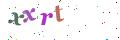English

# Definition of the DC motor

This motor follows linear laws of operation and because of this it is easier to fully exploit its characteristics compared to synchronous or asynchronous motors.

Composition of a D.C. Motor:
The stator is formed by a metal carcass and one or more magnets that create a permanent magnetic field inside the stator. At the rear of the stator are the brush mountings and the brush gear which provide electrical contact with the rotor.The rotor is itself formed by a metal carcass carrying coils which are interconnected at the commutator at the rear of the rotor. The commutator and brush assembly then select the coil through which the electric current passes in the opposite direction.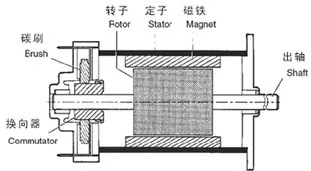Principle of operationWhatever the complexity of the rotor coil windings, once they areenergised, they may be represented in the form of a ferromagneticcylinder with a solenoid wrapped around it.
The wire of the solenoid is in practice the wire bundle located ineach groove of the rotor. The rotor, when energised, then acts as anelectromagnet, the magnetic field following the axis separating the wiresof the solenoid in the direction of the current which flows through them.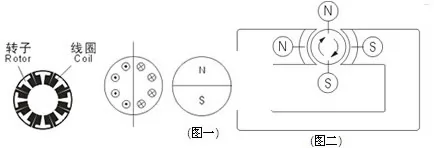The motor, therefore, consists of fixed permanent magnets (the stator) amoving magnet (the rotor) and a metal carcass to concentrate the flux(the motor body).(DRW 1)
(DRW 2)By the attraction of opposite poles and repulsion of like poles, a torque then acts on the rotor and makes it turn. This torque is at a maximum when the axis between the poles of the rotor is perpendicular to the axis of the poles of the stator.As soon the rotor begins to turn, the fixed brushes make and break contact with the rotating commutator segments in turn.The rotor coils are then energised and de-energised in such a way that as the rotor turns, the axis of a new pole of the rotor is always perpendicular to that of the stator. Because of the way the commutator is arranged, the rotor is in constant motion, no matter what its position. Fluctuation of the resultant torque is reduced by increasing the number of commutator segments, thereby giving smoother rotation.By reversing the power supply to the motor, the current in the rotor coils,and therefore the north and south poles, is reversed. The torque which acts on the rotor is thus reversed and the motor changes its direction of rotation. By its very nature, the D.C. motor is a motor with a reversible direction of rotation.

Torque and speed of rotation:
The torque generated by the motor, and its speed of rotation, are dependent on each other.
This is a basic characteristic of the motor ; it is a linear relationship and is used to calculate the no-load speed and the start-up torque of the motor.(DRW 1)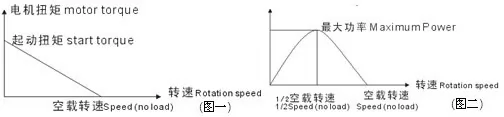The curve for the output power of the motor is deduced from the graph of torque versus speed. (DRW 2)The torque vs. speed and output power curves depend on the supply voltage to the motor.
The supply voltage to the motor assumes continuous running of the motor at an ambient temperature of 20℃ in nominal operational conditions.

It is possible to supply the motor with a different voltage (normally between -50% and + 100% of the recommended supply voltage).If a lower voltage is used compared to the recommended supply the motor will be less powerful.If a higher voltage is used, the motor will have a higher output power but will run hotter (intermittent operation is recommended).

For variations in supply voltage between approximately - 25% to + 50%,the new torque vs. speed graph will remain parallel to the previous one.Its start-up torque and no-load speed will vary by the same percentage (n%) as the variation in supply voltage. The maximum output power is multiplied by (1 +η%)2.

Example : For a 20% increase in supply voltage
Start-up torque increases by 20% ( x 1.2)
No-load speed increases by 20% ( x 1.2)
Output power increases by 44% ( x 1.44)
Torque and supply current: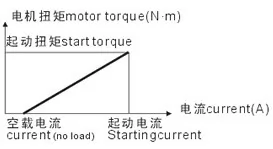This is the second important characteristic of a D.C. motor.It is linear and is used to calculate the no-load current and the currentwith the rotor stationary (start-up current).

The graph for this relationship does not vary with the supply voltage
of the motor. The end of the curve is extended in accordance with the torque and the start-up current.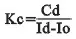This torque constant is such that:：C=Kc(I－Io)   The otational friction torque is Kc. Io.    The torque is therefore expressed as follows :C=Kc. I－Cf   Cf=Kc. Io
Kc = Torque constantr (N.m/A)           C = Torque (N.m)
Cd= Start-up Torque (N.m)                 Cf = Rotational friction torque (N.m)
I = Current (A)                Io = No-load current (A)               Id = Start-up current (A)
The gradient of this curve is called the “torque constant” of the motor.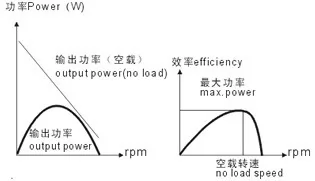Efficiency
The efficiency of a motor is equal to the mechanical output power that it can deliver, divided by the power which it absorbs.The output power and the absorbed power vary in relation to the speed of rotation, therefore the efficiency is also a function of thespeed of the motor.Maximum efficiency is obtained with a given rotational speed greater than 50% of no-load speed.

Temperature rise
The temperature rise of a motor is due to the difference between the absorbed power and the output power of the motor. This difference is the power loss.Temperature rise is also related to the fact that power loss, in the form of heat from the motor, is not rapidly absorbed by the ambient air (thermal resistance). The thermal resistance of the motor can be greatly reduced by ventilation.

Important
The nominal operating characteristics correspond to the voltagetorque-speed characteristics required for continuous operation at an ambient temperature of 20℃. Only intermittent duty is possible outside these operating conditions : without exception, all checks concerning extreme operating conditions must be performed in the actual customer application conditionsin order to ensure safe operation.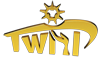NO.1269 Mingshu Road,Jiangshan Industrial Zone, Yinzhou District, NingboZhejiang Province China
You can trust us
We are a professional Manufacturer in China, and we are constantly innovating so that our customers can have better products and services.